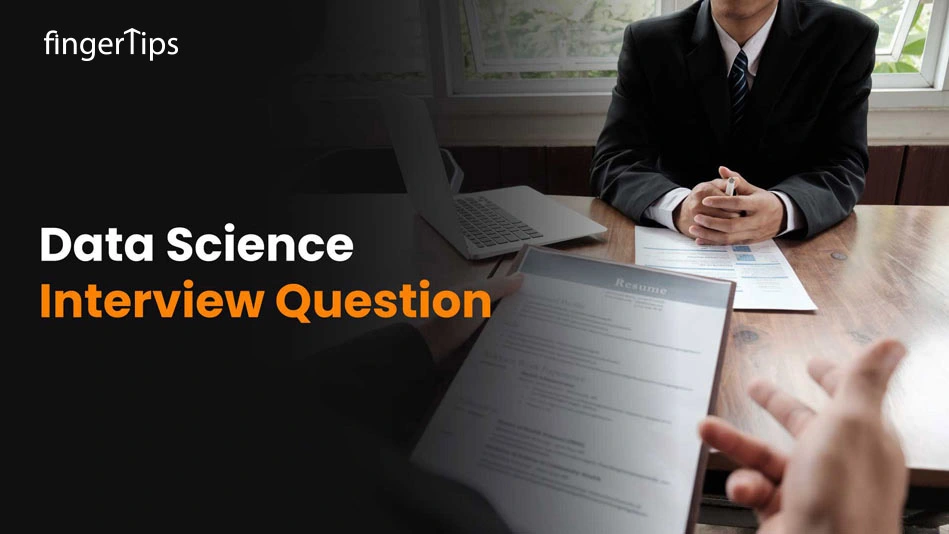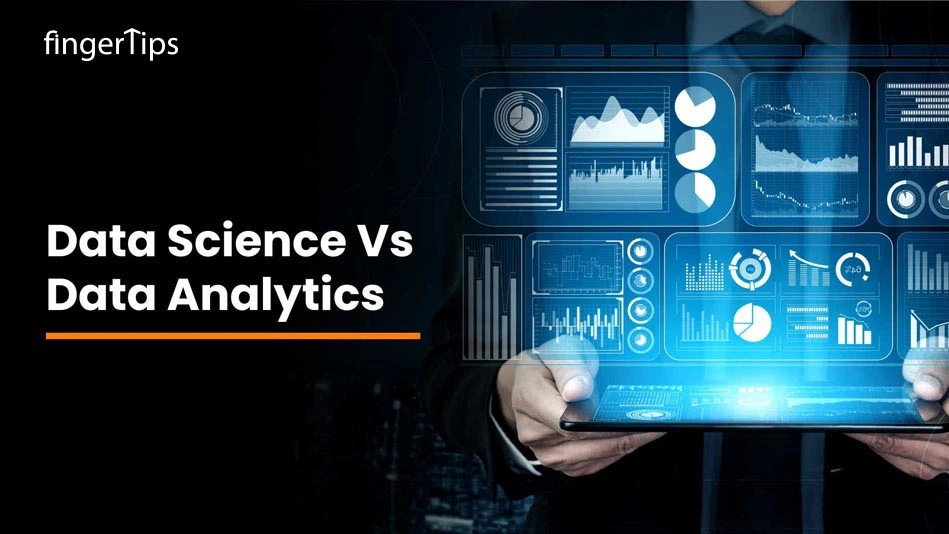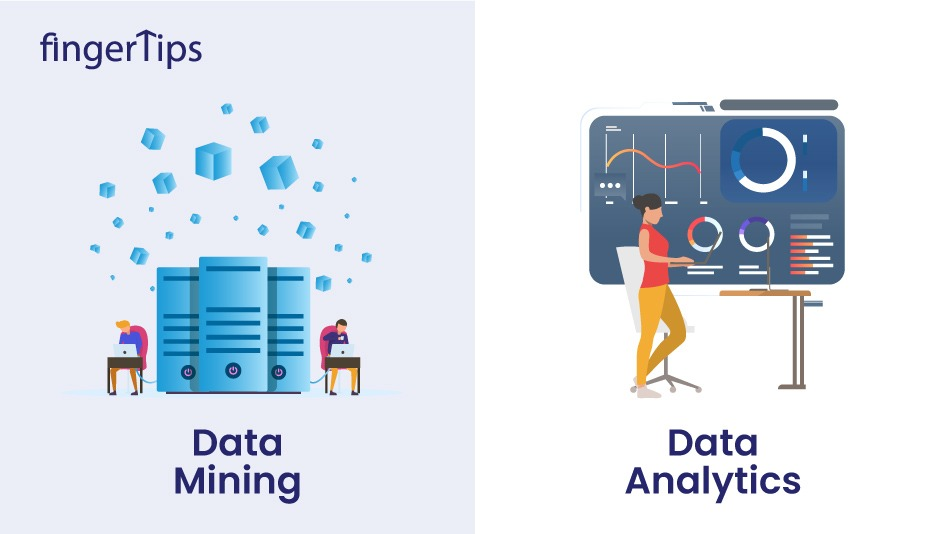Home > Blog > Data Science > Data Science Interview Question For 2023

# Data Science Interview Question For 2023

13 Oct 2022
3000### Related TopicsInterested in this course?## Introduction

Are you planning to move on the path to becoming a data scientist? Or is it, you have finally bagged an interview for it? But you are from a Non-Tech background and want to switch your career entirely from a Non Tech to Data Scientist, So how are you going to prepare for the data scientist interview? We have got you covered!

Data Science interview preparation is very different than any other technical interview due to the nature of the work that a data scientist performs. You may be asked questions about anything ranging from statistics, machine learning, deep learning, and data visualization.

And if you're pursuing a career as a data scientist, you need to be ready to dazzle potential employers with your expertise. You must be able to ace your upcoming data science interview in one go in order to accomplish that. Relax, we have got you covered! To help you clear your interview and get you closer to your dream job, we have compiled the top data science interview questions and answers in one place for your convenience. So, let's have look at the most frequently asked Data Science interview questions for both new and experienced candidates. But before that here's a short explanation of what is Data Science.

## What is Data science?

In simple words, Data Science is making the raw data intelligent by using the concept of Machine Learning and Deep Learning. It analyzes data for actionable insights. Using these insights, we can know the taste of customers and predict the success of the product in the market. "What is data science?" is also one of the most common questions asked by an interviewer, hence you can give this simple explanation. Okay, now let's move on to the important Data Science Interview Questions and answers that may help you crack your data science interview successfully.

## Basic Data Science Interview Questions and Answers

Here is a list of the top technical data science interview questions you may anticipate being asked, along with advice on how to respond to them.

### 1. What is Deep Learning?

Deep Learning is an advanced version of neural networks to make the machines learn from data. In Deep Learning, the neural networks comprise many hidden layers (which is why it is called �deep� learning) that are connected to each other, and the output of the previous layer is the input of the current layer.

### 2. How can we Explain Bias in Data Science?

Bias is an error that takes place in a Data Science model when an algorithm is not strong enough to capture the trends that exist in the data. In simple words, it occurs when the data is very complicated and the algorithm is not able to understand it. As a result, a model is built that makes simple assumptions which may lead to lower accuracy. Linear regression, logistic regression are some of the Algorithms that can lead to high bias.

### 3. What is logistic Regression?

Logistic regression is a predictive analysis. Logistic regression is used to describe data and to explain the relationship between one dependent binary variable and one or more independent variables. It just means a variable that has only 2 outputs, The outcome can either be yes or no.

### 4. Explain logistic Regression by giving an example?

Let's say suppose we want to predict if it will rain or not based on two factors that are temperature and humidity. Here Rain is the dependent variable and Temperature and humidity is the independent variable. Another example of linear regression can be of predicting if the student will pass this exam or not based on the no. of hours he studies.

### 5. What do you understand by linear regression?

Linear regression is a basic and commonly used type of predictive analysis. Linear regression analysis is used to predict the value of a variable based on the value of another variable. The variable you want to predict is called the dependent variable. The variable you are using to predict the other variable's value is called the independent variable.

### 6. What is the Difference Between Data Analytics and Data Science?

Data analytics is just the subset of Data Science that needs basic programming languages. In simple words, it is just analysis of data to make decisions. The main aim here is just to find solutions. Whereas Data Science is a broad technology that includes many subsets like Data Analytics, Data Visualization, Data mining, etc. For it, you need to know advanced programming languages. It not only helps to find solutions but also predict the future of the product with the help of past patterns and insights. Overall the goal of Data Science is to discover meaningful insights from massive datasets.

### 7. What are some Popular Data Science Libraries?

Few of the most popular libraries used for data extraction, cleaning, visualization, and deploying Data science models are

• TensorFlow that supports parallel computing with outstanding library management supported by Google.
• SciPy that mainly uses graphs and charts to solve data manipulation, visualization, differential equations, and multidimensional programming through graphs and charts.
• Pandas which is used to implement the ETL meaning Extracting, Transforming, and Loading the datasets capabilities in business applications.
• Matplotlib That is a free and an open-source. It can be the alternative for MATLAB, which results in better performance and low memory consumption.

### 8. What is statistics?

Statistics is a branch of mathematics where collection, analysis and interpretation of data is involved. And with the help of various tools and techniques in statistics, the raw data becomes meaningful and generates various information for decision-making purposes.

Statistics is very important when it comes to the conclusion of the research. Today, statistical methods are applied in most of the fields that involve decision-making, so that accurate inferences from arranged data can be made.

### 9. What is Hypothesis testing in statistics?

Hypothesis testing in statistics is used to see meaningful results from certain experiments. It helps us to assess the statistical significance of insight just by determining the odds of the results occurring by choice. Here in hypothesis testing, we calculate the p-value after knowing the null hypothesis and if the null hypothesis is true, other values are also determined.
Also if the p-value is less than alpha, the null hypothesis will get rejected, but if it is greater than the alpha, the null hypothesis is accepted.

### 10. What is meant by �P-value�?

P-value in statistics is calculated during the hypothesis testing, also we can say that it is a number that indicates the likelihood of data occurring by a random chance. If a p-value is 0.5 and is less than alpha, then we can conclude that there is a probability of 5% that the experiment results have occurred by chance.

### 11. What is �Descriptive Statistics�?

Descriptive statistics is used to summarize some of the basic characteristics of a data set in a study or experiment.
It is of three main types:

• Distribution: In Distribution we can refer to the frequencies of responses.
• Central tendency: It gives us a measure or the average of each responses.
• Variability: It shows the dispersion of a data set.

### 12. Why R is mostly used in Data Visualization?

For the following reasons, R is frequently used in data visualizations:

• R allows us to make practically any type of graph.
• Lattice, ggplot2, Leaflet, and other libraries are just a few of the many built-in functions in R.
• In comparison to Python, R makes it simpler to personalize graphics.
• R is used for both exploratory data analysis and feature engineering.

### 13. What does NLP stands for?

The term "Natural Language Processing" (NLP) examines how computers use programming to learn a vast amount of textual information. Stemming, Sentimental Analysis, Tokenization, the elimination of stop words are some common examples of Natural Language Processing.

### 14. What does Machine Learning mean and mention some of its types?

Machine Learning is a branch of artificial intelligence that allows machines to learn and develop without being programmed at each and every stage.

Machine learning also analyses data, interprets it, learns from it, and makes the best possible business decisions on the basis of the learning using a set of learning algorithms.
These algorithms get divided into four categories:

• Supervised Learning: This approach can be used to predict future scenarios and gets applied to the learning based on the past data to the new data using labeled
• Decision Trees, Logistic Regression, KNN algorithm, etc., are some of the common supervised learning algorithms.
• Unsupervised learning: This approach refers to a sort of machine learning in which the machine learns from the data without the assistance of a human.
•  Also, unsupervised models can be trained with an unlabeled dataset that is neither classified nor categorized, and the algorithm must process the data without supervision.
• Some common Unsupervised learning algorithms can be K-means Clustering, Apriori Algorithm, etc.
• Semi-Supervised Learning: It is similar to supervised learning let can be trained by both labeled and unlabeled data.
• Reinforcement Learning: It is a branch of machine learning which is about learning the optimal behavior in an environment to obtain a maximum reward.

### 15. What is the Major difference between the Training Set and the Test set?

The Training set is the example given to the model to analyze and learn whereas in the Test Set the accuracy of the hypothesis generated by the model is get tested. Also Training Set is the labeled data used to train the model while in the Test Set we test without labeled data and then verify results with labels.

### 16. What is Artificial Intelligence?

Artificial Intelligence is a method of making a computer-controlled robot or software which thinks intelligently like the human mind. Artificial Intelligence is accomplished by studying the patterns of the human brain and by analyzing the cognitive process.

### 17. What is TensorFlow? And what it is used for?

TensorFlow is an open-source software library initially developed by the Google Brain Team that can be used in Machine learning and neural network research. It is used for data-flow programming.
It makes it so much easier to build certain AI features into applications, including natural language processes and speech recognition.

### 18. What are Neural Networks?

Neural Network is nothing but a series of algorithms that reflects the behavior of the human brain, allowing computer programs to recognize patterns and solve common problems in the field of AI, machine learning, and deep learning.
Real-life examples can be Siri, Alexa, self-driving cars, and even Email spam filter is an example of artificial Intelligence.

## Data Science Interview Tips

There are several Data Science Interview Tips and here we bring some of them:

• Research the role and identify where you will fit
• Get an idea of what the interviewer is looking for
• Always discuss the salary part

## Conclusion

Although the task is challenging for data scientists, it is a lucrative career, and there are many job openings. You may be able to go one step closer to your ideal job by using these data science interview questions. So, get ready for the challenges of interviews and keep up with the details of data science.Fingertips